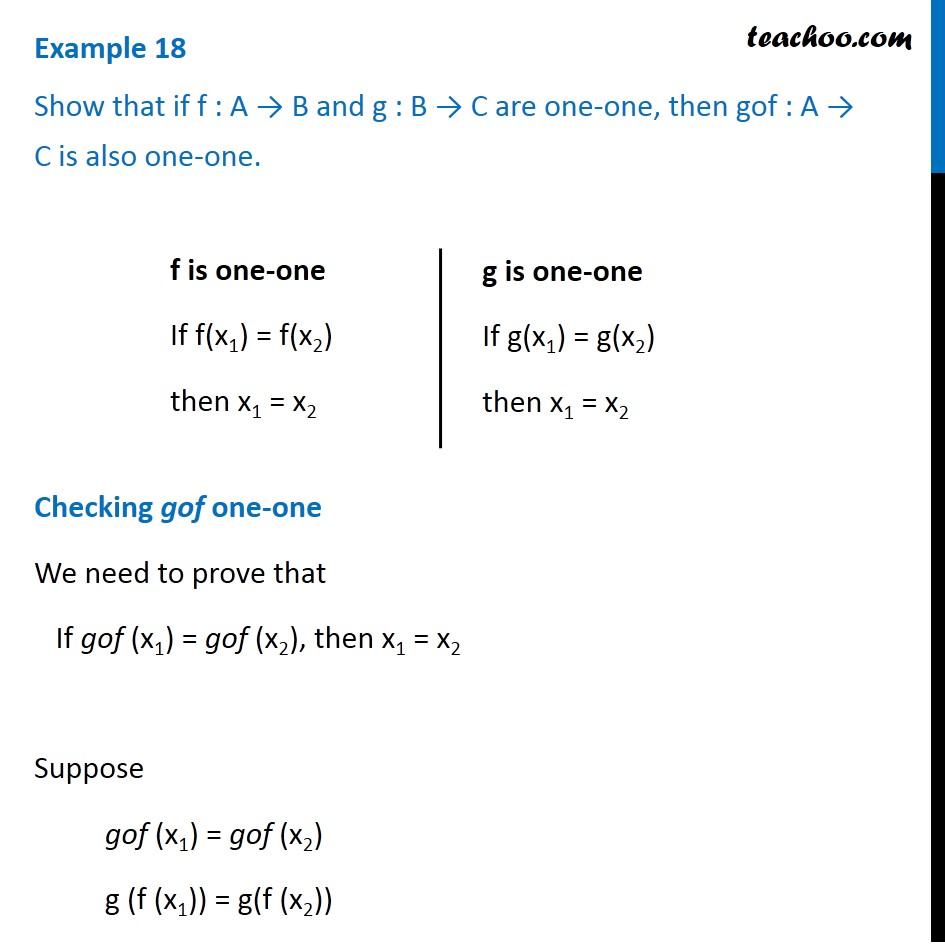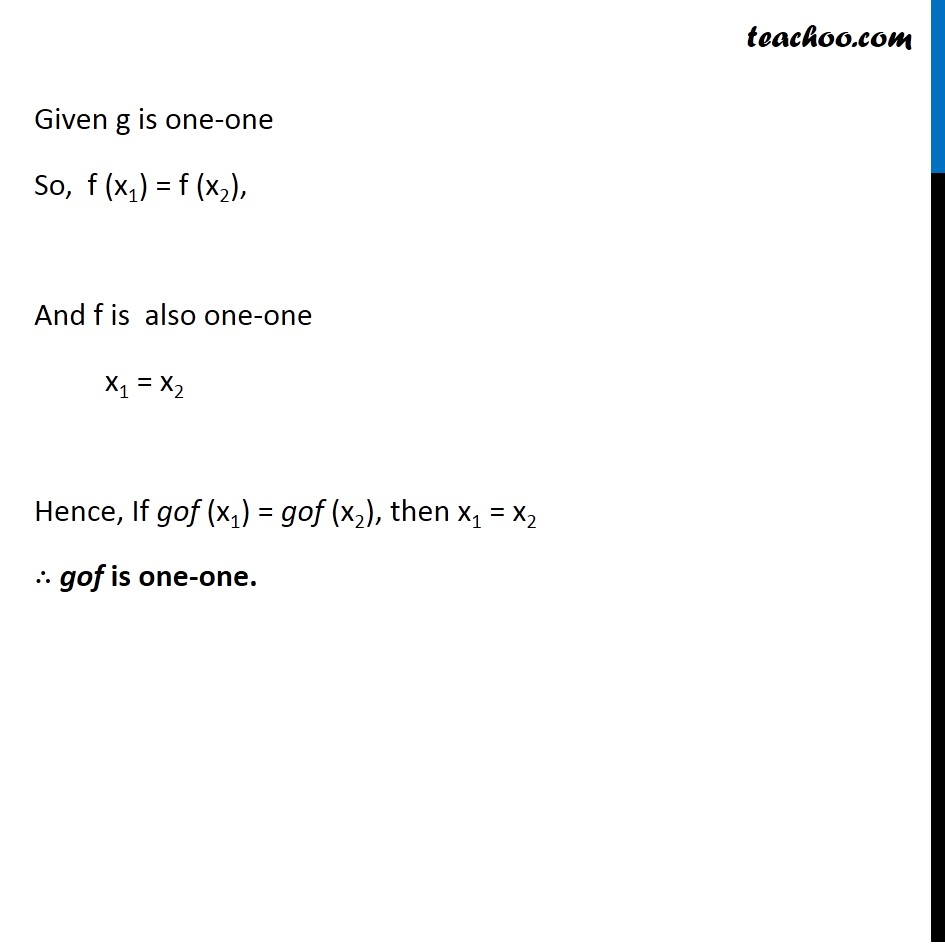Composite functions and one-one onto

Chapter 1 Class 12 Relation and Functions
Concept wiseLearn in your speed, with individual attention - Teachoo Maths 1-on-1 Class

### Transcript

Question 2 Show that if f : A → B and g : B → C are one-one, then gof : A → C is also one-one. Checking gof one-one We need to prove that If gof (x1) = gof (x2), then x1 = x2 Suppose gof (x1) = gof (x2) g (f (x1)) = g(f (x2)) Given g is one-one So, f (x1) = f (x2), And f is also one-one x1 = x2 Hence, gof is one-one.